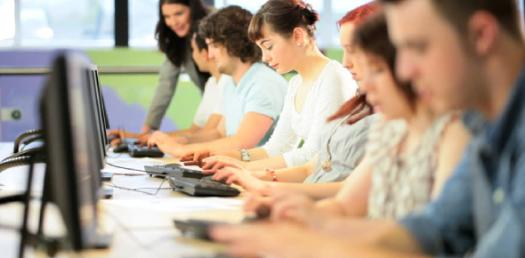# Computer Knowledge Test For Students! Trivia Quiz

10 Questions | Total Attempts: 529SettingsAre you a student taking up computer studies? If you said yes, then you have come to the right place as the test below is designed just for you. Not only will it test you on the basics, but it will also show you a scoop of what type of questions you might get in the assessment tests in class. Do give it a shot!

• 1.
The daily processing of corrections to customer accounts best exemplifies the processing mode of:
• A.

1) Batch processing

• B.

2) Real-time processing

• C.

3) Interactive processing

• D.

4) Multi processing

• E.

5) All of the above.

• 2.
Which is a unit of measurement used with computer systems?
• A.

1) Byte

• B.

2) Kilobyte

• C.

3) Megabyte

• D.

4) Gigabyte

• E.

5) All of the above

• 3.
A character is represented in EBCDIC by
• A.

1) one bit

• B.

2) four bits

• C.

3) eight bits

• D.

4) sixteen bits

• E.

5) None of the above

• 4.
Which is a secondary memory device?
• A.

1) CPU

• B.

2) ALU

• C.

3) Floppy disk

• D.

4) Mouse

• E.

5) None of the above

• 5.
Which is the computer memory that does not forget?
• A.

1) ROM

• B.

2) RAM

• C.

3) PROM

• D.

4) All of the above

• E.

5) None of the above

• 6.
The most common binary code in use today is the 8 bit ASCII code. What do the letters ASCII stand for?
• A.

1) American Standard Code for International Interchange

• B.

2) American Standard Code for Information Interchange

• C.

3) American Standard Code for Intelligence interchange

• D.

4) American Scientific Code for Information Interchange

• E.

5) None of the above

• 7.
A computer derives its basic strength from
• A.

1) Speed

• B.

2) Accuracy

• C.

3) Memory

• D.

4) all of the above

• E.

5) None of the above

• 8.
A collection of eight bits is called
• A.

1) Byte

• B.

2) Word

• C.

3) Record

• D.

4) file

• E.

5) None of the above

• 9.
A BIT represents a
• A.

1) Decimal digit

• B.

2) Octal digit

• C.

3) Binary digit

• D.

• E.

5) none of these

• 10.
Which can read data and convert them to a form that a computer can use?
• A.

1) Logic

• B.

2) Storage

• C.

3) Control

• D.

4) Input device

• E.

5) Output device

Related TopicsBack to top